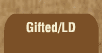Mathematics and Dyslexia

Tips for Learning the Times Tables

Tools for the Times Tables & Workbook

Home > Math > times tables practice > Threes times tables (low half, in order)

## The Threes Times Tables

The digits to numbers that you can divide by three always add up to a number that you can divide by three. For 12, 2 + 1 is 3; for 18, 8 +1 is 9; for 51, 5 +1 is 6. (When you have to reduce fractions, math problem makers love to put 51 in there. Now you know! Three goes into it!!!)

Remember, an odd times an odd number gives you an odd number. If there's an even number there, the answer will be even.

1. 3 x 0=
2. 3 x 1=
3. 3 x 2=
4. 3 x 3=
5. 3 x 4=
6. 3 x 5=
7. 5 x 3=
8. 4 x 3=
9. 3 x 3=
10. 2 x 3=
11. 1 x 3=
12. 2 x 3=
13. 3 x 3=
14. 4 x 3=
15. 5 x 3=